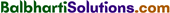By going through these Maharashtra State Board 12th Science Chemistry Notes Chapter 6 Chemical Kinetics students can recall all the concepts quickly.

## Maharashtra State Board 12th Chemistry Notes Chapter 6 Chemical Kinetics

→ For a reaction, aA + bB → cC + dD
Average rate = $$-\frac{1}{a} \frac{\Delta[\mathrm{A}]}{\Delta t}=-\frac{1}{b} \frac{\Delta[\mathrm{B}]}{\Delta t}=-\frac{1}{c} \frac{\Delta[C]}{\Delta t}=-\frac{1}{d} \frac{\Delta[\mathrm{D}]}{\Delta t}$$

→ Rate law : Rate = k [A]a x [B]b

→ k = $$\frac{2.303}{t}$$ log10 $$\frac{[\mathrm{A}]_{0}}{[\mathrm{~A}]_{\mathrm{t}}}$$ (For first order reaction)→ t1/2 = $$\frac{0.693}{k}$$ (For first order reaction)

→ k = $$\frac{[\mathrm{A}]_{0}-[\mathrm{A}]_{t}}{t}$$ (For zero order reaction)

→ t1/2 = $$\frac{[\mathrm{A}]_{0}}{2 k}$$(For zero order reaction)

→ k = Ae-Ea/RT (Arrhenius equation)

→ log10k =log10A – $$\frac{E_{a}}{2.303 R \mathrm{~T}}$$→ log10$$\frac{k_{2}}{k_{1}}=\frac{E_{a}\left(T_{2}-T_{1}\right)}{2.303 R \times T_{1} \times T_{2}}$$